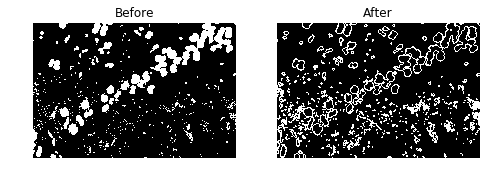Notebook

# Digital Image Processing

In :
%run load_lib_ch3.py


## Chapter 3: Morphological Image Processing

In this chapter, the most common morphological operations will be demonstrated. Morphological Image Processing is a term describing image processing methods that do not rely on the pixels' values but rather on their relative ordering. They are typically used for binary images and in some cases also for grayscale images. For a morphological function on an image $M(f(x,y)) = g(x,y)$, the relationship $M(f(x+a,y+b))=g(x+a,y+b)$ is also true. This means the Morphological operations are shift-invariant.

In :
plt.imshow(img2)
plt.axis('off')
plt.show()
binary_img = fn.otsu(img2)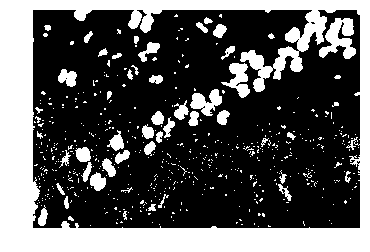They apply a structuring element at all possible locations in an image. It is a kernel matrix with binary values. The most common kernels are All-ones, cross, eclipse/round, but other kernels could be used for detection of a particular pattern.

In :
kernel = np.array([
[0,0,1,0,0],
[0,0,1,0,0],
[1,1,1,1,1],
[0,0,1,0,0],
[0,0,1,0,0]
], dtype=np.uint8)

In :
stuctural_element_plot(kernel)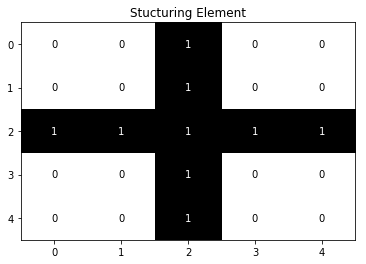The mathematical definition of a morphological operation for binary images is as follows:

Suppose that $F$ is the set of $x$,$y$ coordinates of the input image, and that $K$ is the set of coordinates for the kernel. Let $K_{xy}$ denote the translation of $K$ so that its origin is at coordinates $x$,$y$.
Then the morphological operation of $F$ by $K$ is simply the set of all points $(x,y)$ such that the conditions are met.

The typical morphological functions that the structural element could be used for are:

• Dilation: The condition is that the intersection of $K_{xy}$ with $F$ is non-empty. It makes the white parts grow.
In :
dilation = cv2.dilate(binary_img,kernel,iterations = 1)
compare_result_bw(binary_img, dilation)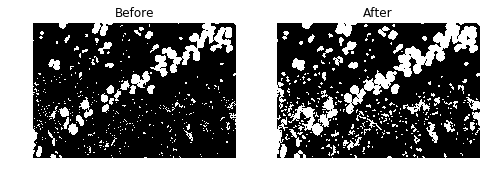• Erosion: The condition is that $K_{xy}$ is a subset of $F$. It makes the white parts shrink
In :
erosion = cv2.erode(binary_img,kernel,iterations = 1)
compare_result_bw(binary_img, erosion)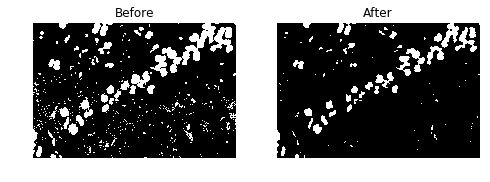• Opening: Is erosion followed by dilation. It can be used remove small white particles.
In :
opening = cv2.morphologyEx(binary_img, cv2.MORPH_OPEN, kernel)
compare_result_bw(binary_img, opening)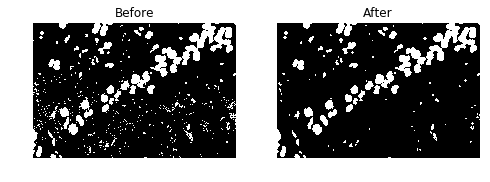• Closing: Is dilation followed by erosion. It can be used to remove small black particles.
In :
closing = cv2.morphologyEx(binary_img, cv2.MORPH_CLOSE, kernel)
compare_result_bw(binary_img, closing)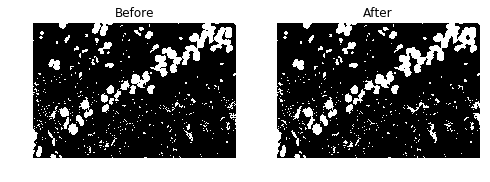• Gradient: The difference between the dilation and erosion of an image. Used for edge detection.
In :
gradient = cv2.morphologyEx(binary_img, cv2.MORPH_GRADIENT, kernel)